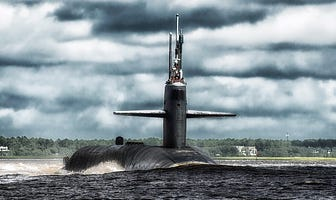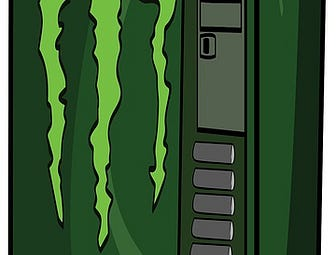My investment approach is simple but not easy: Research companies and determine whether they’re ‘wonderful’ firms worthy of being held for a long period…Key Statistics EV/EBIT = 26.37x ROE = 29.72% Debt/Equity = 79% FCF Yield = 2.32% Dividend Yield = 1.24% Market Cap = \$31.87 billion The Company…Key Statistics EV/EBIT = 14.84x ROE = 69.84% Debt/Equity = 116% FCF Yield = 3.03% Dividend Yield = 1.2% Market Capitalization = \$29.06 billion The…Key Statistics EV/EBIT = 26.25x ROE = 44.5% Debt/Equity = 145% FCF Yield = 1.81% Dividend Yield = 1.04% Market Capitalization = \$68.87 billion The…Performance I closed 2022 down 13.52% in the active account tracked on this substack. Global stocks, as measured by the VT ETF, ended the year down…

### December 2022Disclosure: I am longKey Statistics EV/EBIT = 22.72x ROE = 17.89% Debt/Equity = 40% FCF Yield = 2.04% Dividend Yield = 1.02% Market Capitalization = \$112.97 billion The…I purchased General Dynamics on October 12th, 2020. I still hold it. This is my write up from October 12th, 2020. Like my purchase of Charles Schwab at…Key Statistics EV/EBIT = 31.17x ROE = 18.55% Debt/Equity = 0% FCF Yield = 1.29% Dividend Yield = 0% Market Capitalization = \$52.81 billion The Company…I wrote the below about Charles Schwab (SCHW) on 9/21/20. This was when I was first getting into quality investing - so the logic & checklist isn’t as…Key Statistics EV/EBIT = 30.70x ROE = 37.71% Debt/Equity = 136% FCF Yield = 1.67% Dividend Yield = 1.1% Market Capitalization = \$85.83 billion The…Key Statistics EV/EBIT = 29.04x ROE = 15.46% Debt/Equity = 117% FCF Yield = 1.69% Dividend Yield = 1.77% Market Capitalization = \$22.47 billion The…